# Monday, March 27th

Beauty of Spring (Ernesto Cortazar - Climbing a Tree) (1).mp3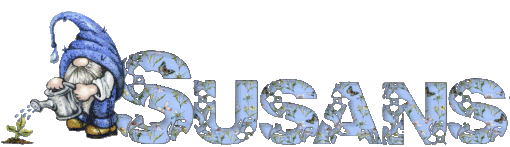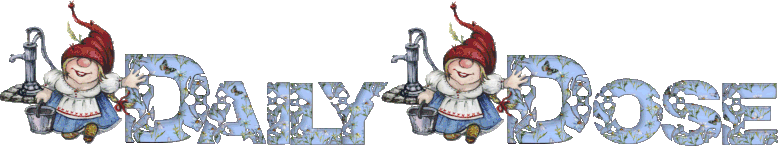Susan Kistler, Creator-Publisher
Since 2001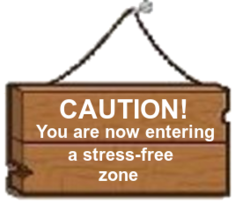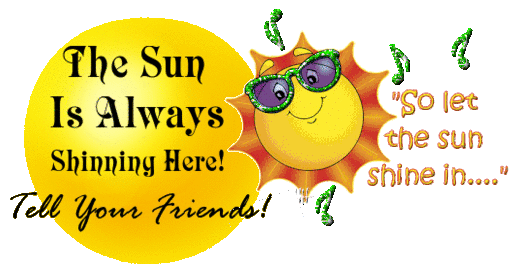Today, March 27th is the 86th day of the year
(87th in leap years) in the Gregorian calendar;
279 days remain until the end of the year.

In 1964 The Beatles' single "can't Buy Me Love" was
released in the U.S. becoming their fourth
consecutive #1 hit.

In 1964 the Good Friday earthquake, the most
powerful earthquake recorded in North American
history at a magnitude of 9.2 strikes South Central
Alaska, killing 125 people and inflicting massive
damage to the city of Anchorage.^^^^^^^^^^^^^^^^^^^^^^^^^^^^^^^^^^^^^^^^^^^^^^^^^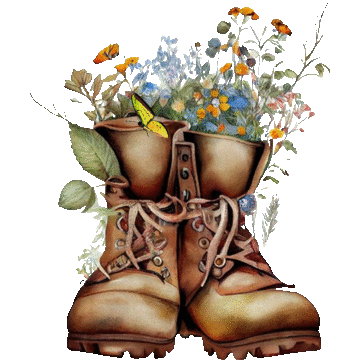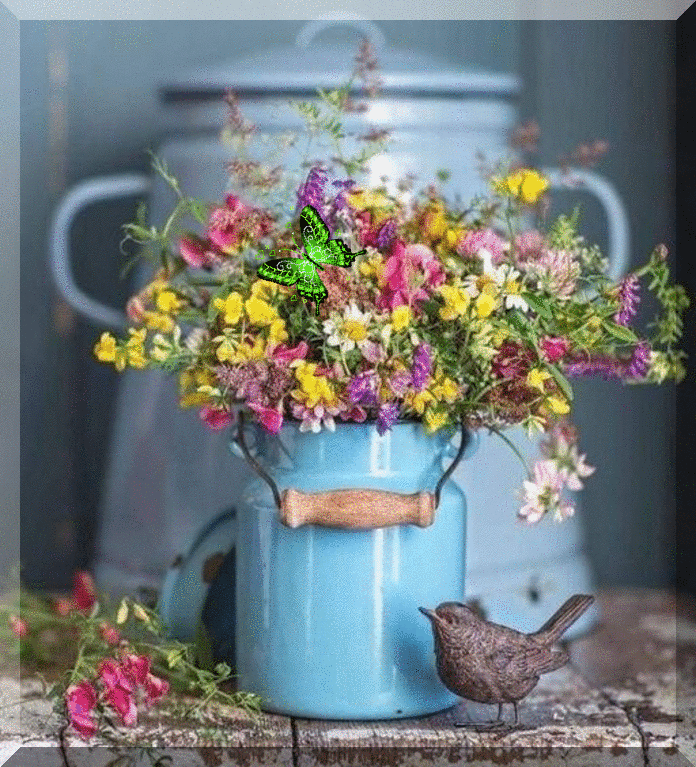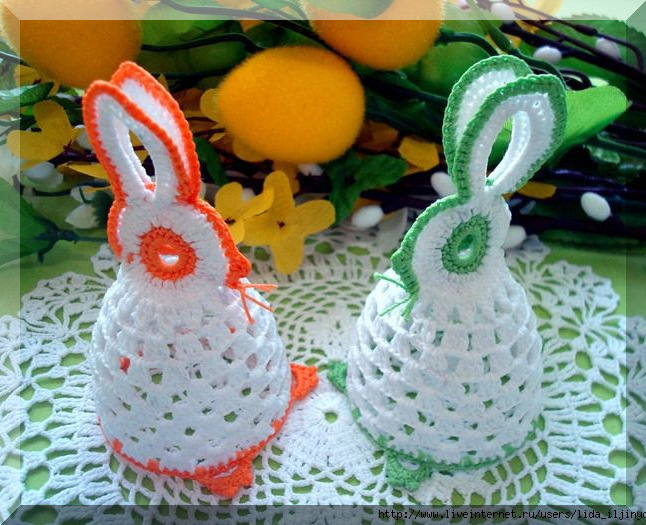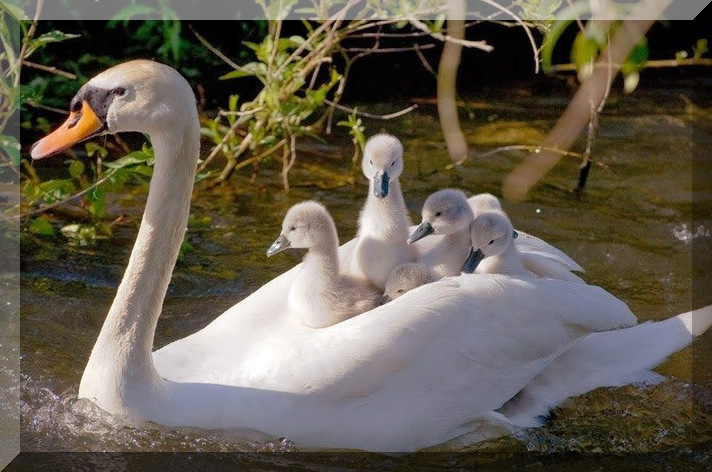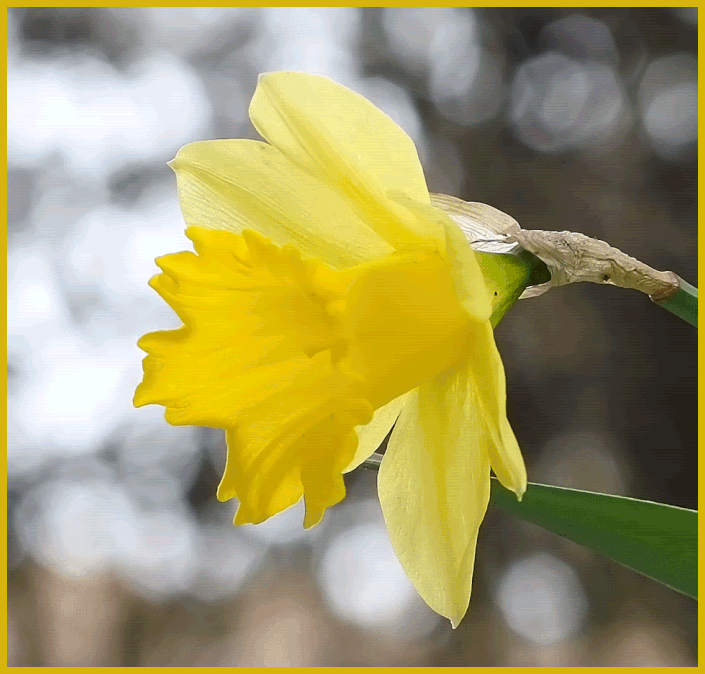^^^^^^^^^^^^^^^^^^^^^^^^^^^^^^^^^^^^^^^^^^^^^^^^^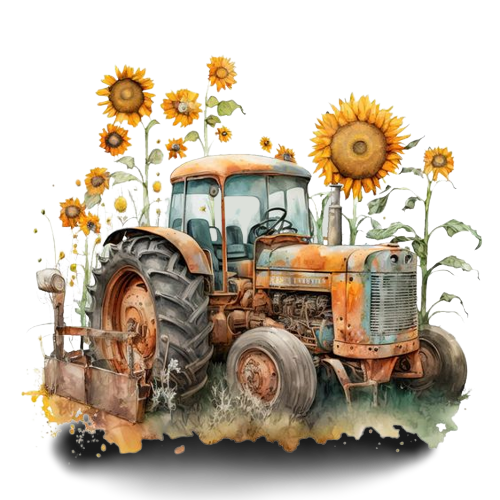^^^^^^^^^^^^^^^^^^^^^^^^^^^^^^^^^^^^^^^^^^^^^^^^^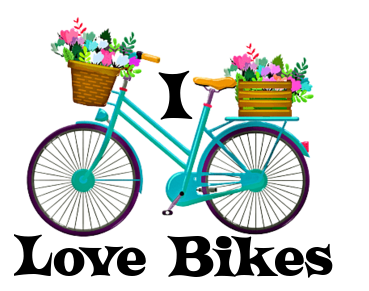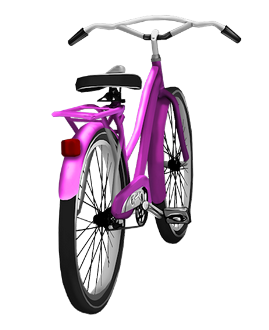^^^^^^^^^^^^^^^^^^^^^^^^^^^^^^^^^^^^^^^^^^^^^^^^^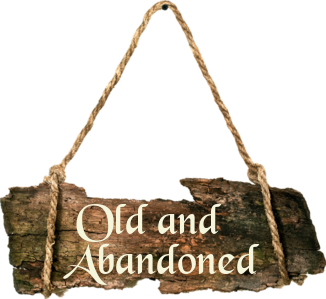I did not know they used to
wallpaper ceilings?

^^^^^^^^^^^^^^^^^^^^^^^^^^^^^^^^^^^^^^^^^^^^^^^^^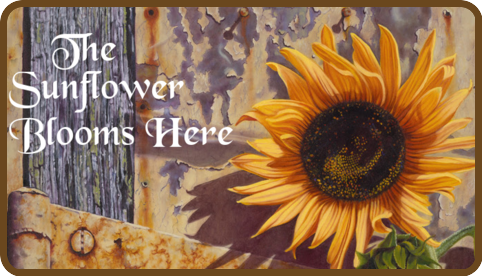^^^^^^^^^^^^^^^^^^^^^^^^^^^^^^^^^^^^^^^^^^^^^^^^^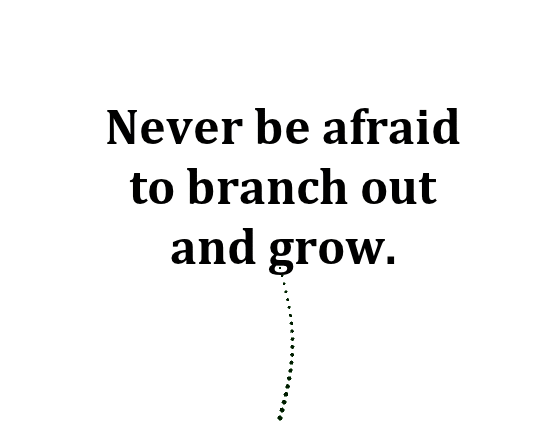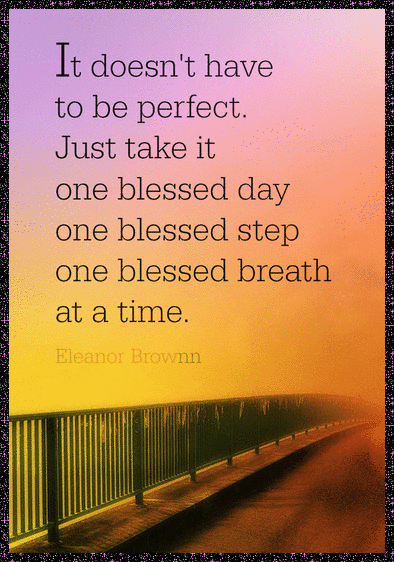^^^^^^^^^^^^^^^^^^^^^^^^^^^^^^^^^^^^^^^^^^^^^^^^^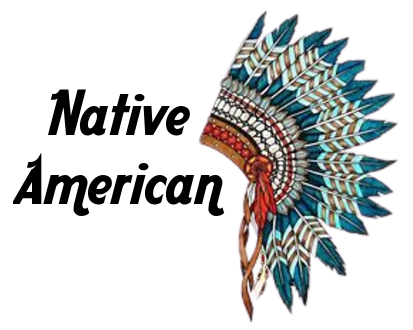^^^^^^^^^^^^^^^^^^^^^^^^^^^^^^^^^^^^^^^^^^^^^^^^^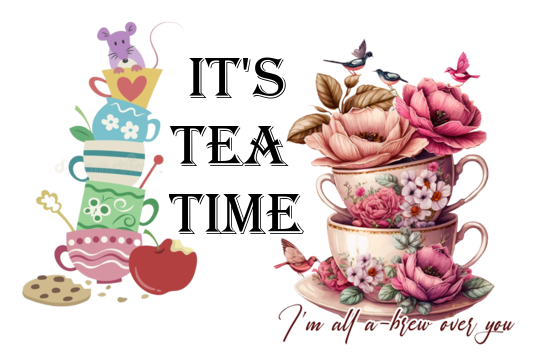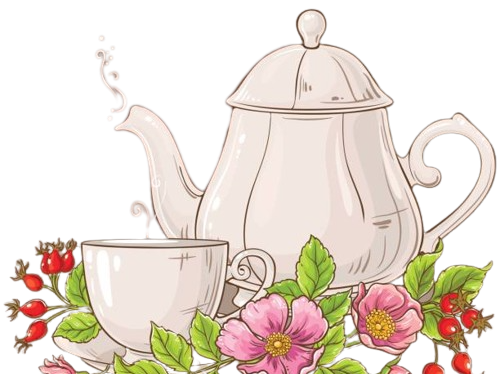^^^^^^^^^^^^^^^^^^^^^^^^^^^^^^^^^^^^^^^^^^^^^^^^^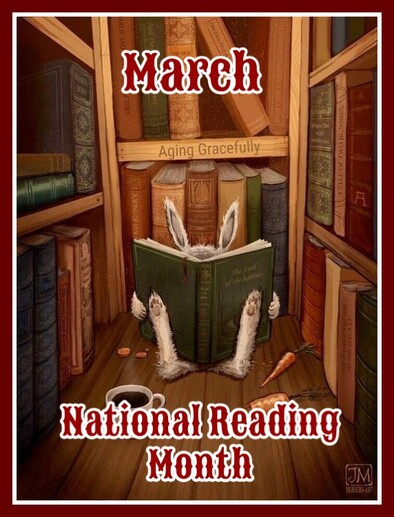In honor of Dr Suess' birthday, March is
designated as Nation Reading Month - a month
to motivate Americans of all ages to read every
day. Reading is fun and has many benefits,
regardless of your age. It's a key component
of education and professional development.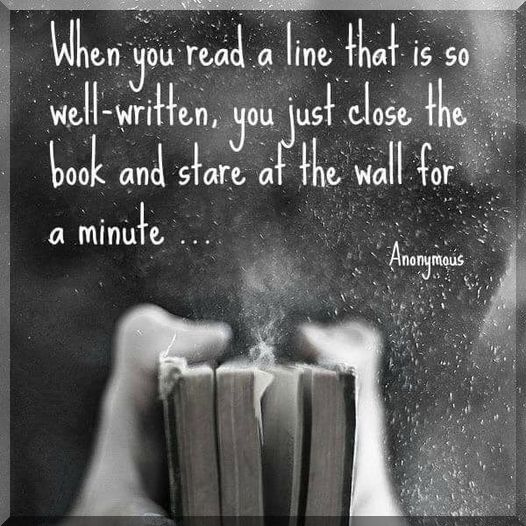^^^^^^^^^^^^^^^^^^^^^^^^^^^^^^^^^^^^^^^^^^^^^^^^^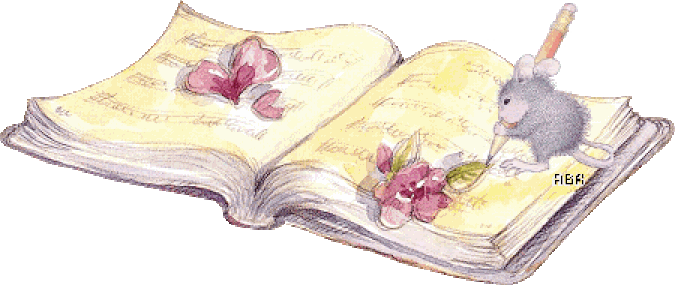^^^^^^^^^^^^^^^^^^^^^^^^^^^^^^^^^^^^^^^^^^^^^^^^^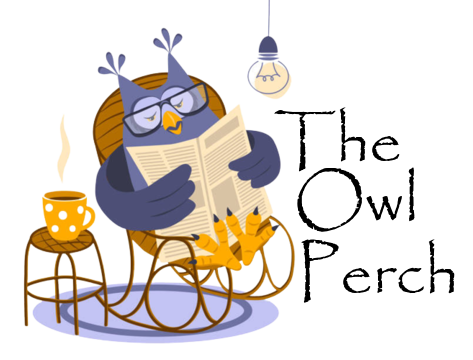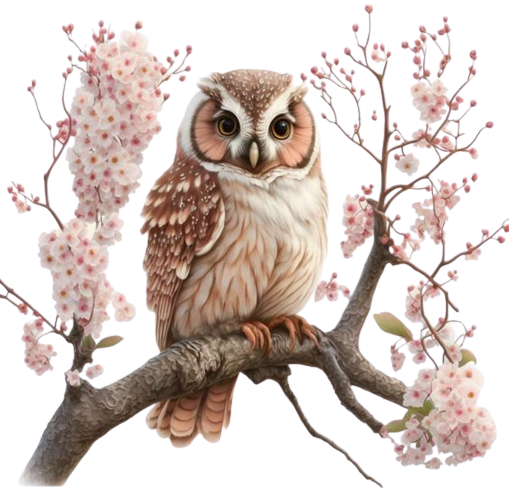^^^^^^^^^^^^^^^^^^^^^^^^^^^^^^^^^^^^^^^^^^^^^^^^^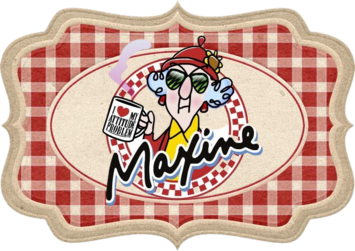^^^^^^^^^^^^^^^^^^^^^^^^^^^^^^^^^^^^^^^^^^^^^^^^^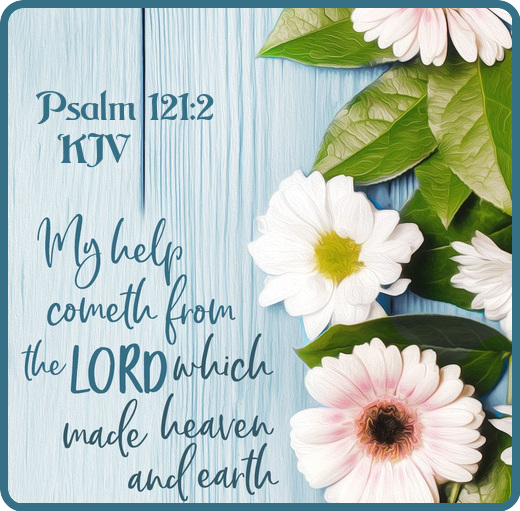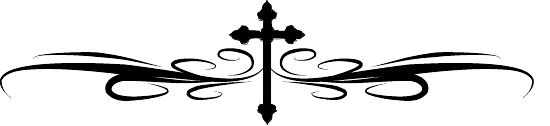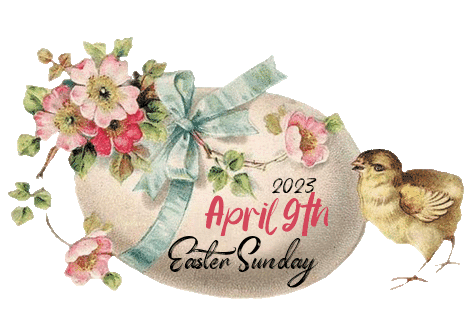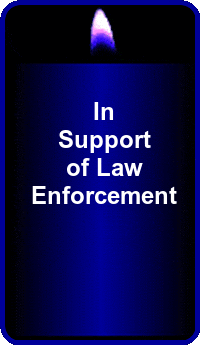I have taken time to include on my pages below.
I will appreciate if you do!
Thank you.Yes, things end,

but memories

last forever ....

Hold tight

to those ...Sharing a wonderful blog/website
https://tattysthingies.blogspot.com/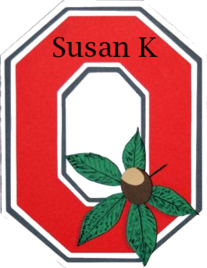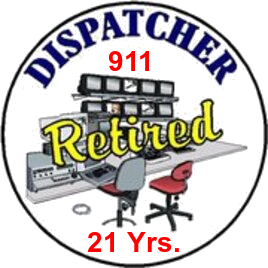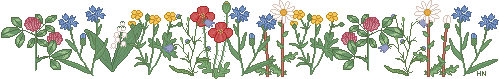Hello, and I would like to let you know that I do accept
donations to help pay for this website. Donations do help
me a lot and are very appreciated. The donate button above
will lead you to PayPal, a safe and easy way to donate.
Not comfortable with PayPal, simply email me and I can
send you my address for mailing. Thank you.The below site will help animals and others. Please
do visit and see how you can help by "just clicking"!
It only takes a minute. I used to have this on my
pages and quit for one reason or another and I
very much wanted to do it again. We all CAN make
a difference. VISIT TODAY!The below map shows
location of visitors